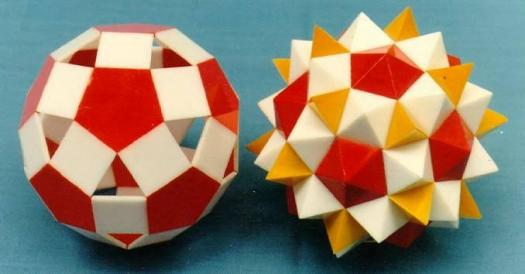# What Do You Know About Polyhedron Models?

Approved & Edited by ProProfs Editorial Team
The editorial team at ProProfs Quizzes consists of a select group of subject experts, trivia writers, and quiz masters who have authored over 10,000 quizzes taken by more than 100 million users. This team includes our in-house seasoned quiz moderators and subject matter experts. Our editorial experts, spread across the world, are rigorously trained using our comprehensive guidelines to ensure that you receive the highest quality quizzes.
A
Community Contributor
Quizzes Created: 145 | Total Attempts: 38,511
Questions: 10 | Attempts: 130SettingsA polyhedron model is described as a physical representation of a polyhedron, made out of a cardboard, panel material, wood board, plastic board or, occasionally, with a solid material. The construction of polyhedron models has now become a common mathematical practice. To learn more, take this short quiz.

• 1.

### When constructing the model, what does the size depicts?

• A.

Durability

• B.

Material

• C.

Height

• D.

Strength

B. Material
Explanation
The size of a model typically represents the material used to construct it. The material choice is important as it determines the overall quality, appearance, and durability of the model. Different materials have different properties and characteristics, which can greatly impact the model's performance and longevity. Therefore, considering the size of the model helps in understanding the material used and its implications on the overall construction.

Rate this question:

• 2.

### What is the folding of a pattern called?

• A.

Net

• B.

Kite

• C.

Folder

• D.

Tower

A. Net
Explanation
The folding of a pattern is called a "net". A net is a two-dimensional representation of a three-dimensional object that can be folded to form the object. It shows all the faces of the object and how they are connected. In the context of patterns, a net represents the flat design that can be folded to create the desired shape or structure.

Rate this question:

• 3.

### From what is the folding of a pattern formed?

• A.

Single sheet of cardboard

• B.

Single sheet of paper

• C.

Double sheets of cardboard

• D.

Multiple sheets of cardboard

A. Single sheet of cardboard
Explanation
The folding of a pattern is formed from a single sheet of cardboard. This means that the pattern is created by manipulating and bending a single piece of cardboard, rather than using multiple sheets or a different material like paper.

Rate this question:

• 4.

### Which factors determine the selection of colors?

• A.

Physical appearance of the polyhedron

• B.

Structure of the polyhedron

• C.

Geometric understanding of the polyhedron

• D.

Algebraic representation of the polyhedron

C. Geometric understanding of the polyhedron
Explanation
The selection of colors for a polyhedron is determined by the geometric understanding of the polyhedron. This means that the colors chosen should be based on the shape, angles, and proportions of the polyhedron in order to accurately represent its geometry. The physical appearance, structure, and algebraic representation of the polyhedron may be important considerations, but ultimately it is the geometric understanding that guides the selection of colors.

Rate this question:

• 5.

### Which of these is an example of complex models?

• A.

Gyrations

• B.

Uniformity

• C.

Stellations

• D.

Cryptography

C. Stellations
Explanation
Stellations are an example of complex models. Stellations involve extending the edges of a polyhedron to create new faces and vertices, resulting in a more intricate and complex shape. This process adds complexity and detail to the original polyhedron, making stellations a clear example of complex models.

Rate this question:

• 6.

### How many polygons are inside the complex model?

• A.

Hundreds

• B.

Thousands

• C.

Tens of thousands

• D.

Dozens

A. Hundreds
Explanation
The correct answer is "Hundreds". This suggests that there are multiple polygons inside the complex model, but not enough to reach the thousands or tens of thousands. It implies that the model is relatively complex, but not excessively so.

Rate this question:

• 7.

### Which of these is an example of stellated octahedron?

• A.

Merkaba religious symbol

• B.

Merkaba architectural design

• C.

Raymond design

• D.

Rhiwana religious symbol

A. Merkaba religious symbol
Explanation
The Merkaba religious symbol is an example of a stellated octahedron. The Merkaba is a three-dimensional star shape that consists of two interlocking tetrahedrons, which are both stellated octahedra. It is often associated with spirituality and is believed to have protective and transformative properties.

Rate this question:

• 8.

### How many polyhedra do we have?

• A.

75

• B.

80

• C.

85

• D.

90

A. 75
Explanation
The question is asking for the number of polyhedra that we have. Out of the given options, the correct answer is 75.

Rate this question:

• 9.

### Which software is used to print nets?

• A.

• B.

Stella

• C.

Aspen Hysis

• D.

B. Stella
Explanation
Stella is the software used to print nets.

Rate this question:

• 10.

### How many polyhedral compounds do we have?

• A.

5

• B.

6

• C.

7

• D.

8Back to top